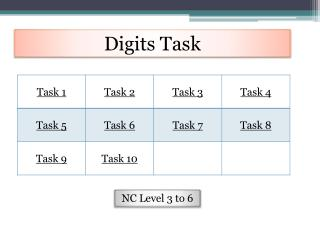DownloadDownload PresentationDownload Presentation- - - - - - - - - - - - - - - - - - - - - - - - - - - E N D - - - - - - - - - - - - - - - - - - - - - - - - - - -
##### Presentation Transcript

1. Digits Task NC Level 3 to 6

2. Digits Task 1 Use these cards to make true statements. Like this one: Can you find a way to use all the cards in one statement? Can you find all the possible ways to use the cards? http://nrich.maths.org/175 Home

3. Digits Task 2 You have a set of digits from 0 to 9 Can you arrange these digits in the ten boxes to the right to make two-digit numbers as close to the targets as possible? You may use each digit once only. http://nrich.maths.org/6343 Home

4. Digits Task 3 You have 2 sets of digits from 0 to 9 Can you arrange these digits in the boxes to the right to make four-digit numbers as close to the targets as possible? You may use each digit once only. http://nrich.maths.org/6342 Home

5. Digits Task 4 Using a grid of four "boxes“, You must choose four different digits from 1−9 and put one in each box. For example: This gives four two-digit numbers: 52 (reading along the 1st row) 19 (2nd row) 51 (left hand column) 29 (right hand column) In this case their sum is 151 . Your challenge is to find four different digits that give four two-digit numbers which add to a total of 100. How many ways can you find of doing it? http://nrich.maths.org/1130 Home

6. Digits Task 5 Jane wants to create a six-digit number for her padlock. She writes down two digits and each digit she writes after these is the sum of the previous two digits. How many six-digit numbers could she create in this way? (A number may not start with the digit zero). http://nrich.maths.org/6773 Home

7. Digits Task 6 This represents the multiplication of a 4-figure number by 3 The whole calculation uses each of the digits 0−9 once and once only. The 4-figure number contains three consecutive numbers, which are not in order. The third digit is the sum of two of the consecutive numbers. The first, third and fifth figures of the five-digit product are three consecutive numbers, again not in order. The second and fourth digits are also consecutive numbers. Can you replace the stars in the calculation with figures? http://nrich.maths.org/1129 Home

8. Digits Task 7 Find the sum of all the three-digit numbers which only have odd digits. http://nrich.maths.org/738 Home

9. Digits Task 8 What is the sum of all the three-digit whole numbers? http://nrich.maths.org/683 Home

10. Digits Task 9 If you wrote all the possible four digit numbers made by using each of the digits 2, 4, 5, 7 once, what would they add up to? http://nrich.maths.org/853 Home

11. Digits Task 10 What is the largest possible five-digit number divisible by 12 that you can make from the digits 1, 3, 4, 5 and one more digit? 5 3 4 ? 1 http://nrich.maths.org/559 Home